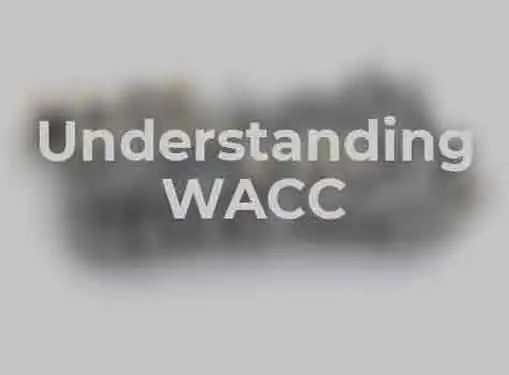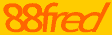# Understanding WACC

Written by Bobby Jan for Gaebler Ventures

Since many analysts use the weighted average cost of capital (WACC) to discount cash flow, it is important to at least understand how WACC works.

Before reading this article on the weighted average cost of capital (WACC), you should get a basic understanding of the discounted cash flow by reading the article Understanding Discounted Cash Flow.WACC helps determine the cost of a firm's capital by weighting each category of capital.

WACC takes into account all categories of capital, including common stocks, preferred stocks, bonds, and other debts. Generally speaking, all categories of capital are either in the form of equity or debt. The following equation is a simple demonstration of how WACC is calculated:

WACC= (cost of equity) * (market value of equity) / (market value of equity plus debt) + (cost of debt) * (market value of debt) * (1 - corporate tax rate) / (market value of equity plus debt).

So how do we derive each component of the equation? Here are the drivers of the WACC formula:

• Market value of equity - total shares multiplied by value per share.
• Market value of debt - often, the value of debt is hard to determine for nonpublic companies. The market value of debt is often close to the book value of debt so the book value of debt could be used as an approximation.
• Total market value of equity plus debt - add the previous two components together.
• The cost of equity - could be determined by using the capital asset pricing model (CAPM).
• The cost of debt - could be determined by using yield rates on bonds and interest rates for bank loans.

Understanding how to break the equation above into more precise components can be quite complicated. By averaging the cost of capital, you can have a better idea of how much each dollar cost to finance a business, which is why may professional use WACC as the discount rate.

Cheng Ming (Bobby) Jan is an Economics major at the University of Chicago who has a strong interest in entrepreneurship and investing.

## Conversation Board

Know a few things about WACC that we didn't cover? We welcome all comments and questions.